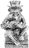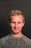### Avg and sum in the same table

Hi

I have a DB of my students. It has different items (with different weighs in the final mark) for the marks. I have a table with marks for each student but I can`t calculate the avg and then the sum of the marks in the same table:

Student

Item 1 mark = Avg(mark 1, mark2, ...)

mark 1

mark 2

...

Item 2 mark = Avg(mark 1, mark2, ...)

mark 1

mark 2

...

Final mark = mark item1 + mark item 2 + ....

I'm grouping by items and calculating the mark for each item but, How can I calculate the sum of the marks to obtain the final mark for each student?Assuming you have a table for Students and a table for Items, you could create a formula field in the Student table.

Field Mark Sum would have a formula sum(Item.Mark)

Hi and thx
I going creating individual marks for each item (at different dates). At the end of a term i need to calculate final mark. The mark for each item (as a avg of diffrent marks for the item) is calculated for the program, grouping by item and choosing avg for the mark column. Each item have a diferent weigh in the final mark.
If I sum all marks get a diferent result (the sum of all marks). I need sum just the heads of the group , but I don't know how can I acces to it.If I understand correctly, In the items table I would have a field for "Items" and a field for "Item Type" as a choice field. The records would be the marks you have for the "Items" and you would also choose "Item Type". This way you can sum all "Items" and you can also sum "Items" where "Item Type" is = choice 1, choice 2, choice n.

Formulas in the Student table

To sum all "Items" - sum(Items.Item)

To sum each "Item Type" - sum(Items[ItemType = 1].Item)

To sum each "Item Type" - sum(Items[ItemType = 2].Item)

To sum each "Item Type" - sum(Items[ItemType = n].Item)In the "Items" table you would have formula fields for each "Item Type".

if ItemType = 1 then Item end

if ItemType = 2 then Item end

.

.

if ItemType = n then Item end

You can group by "Item Type" and average those groups.

Thx a lot for your time.

I knew this way, but it is a bad solution for my app becouse I have different courses with different items (and different number of items as well). It force me to a many lines of code, and a complicated way to show different and more or less items depending on the course. In others words, I think that is a static solution.
Maybe exits another solution in the printer view for instance. I'm goning to investigate it.

Thx againHello,

I'm a TA in one of the private schools in Germany (Internatgymnasium Schloss Torgelow Private School) and I'm new to Ninox. I wanted to calculate the final average grade for each student and I couldn't do it. Everything worked fine with the Attendance record though. What are the possible ways to calculate the sum of the marks and get an average grade from half of the class?